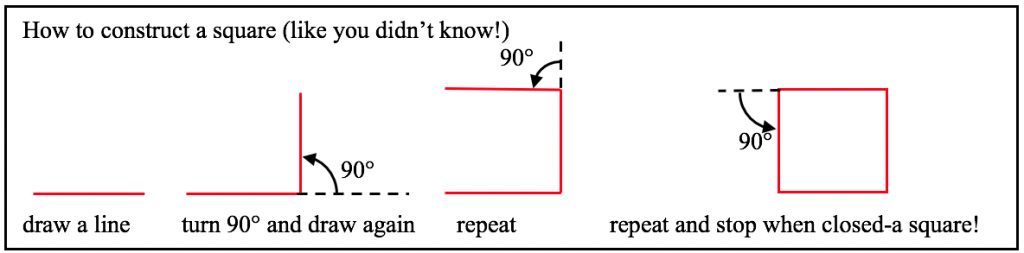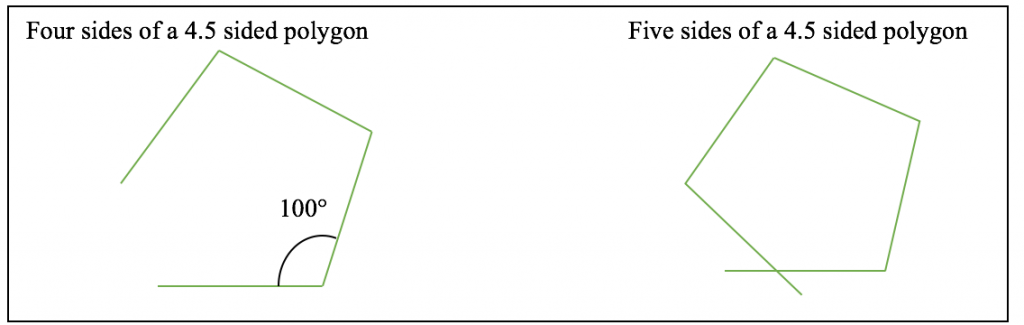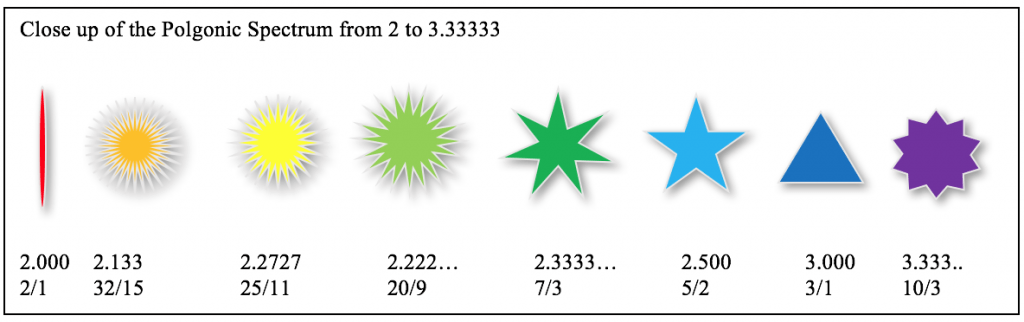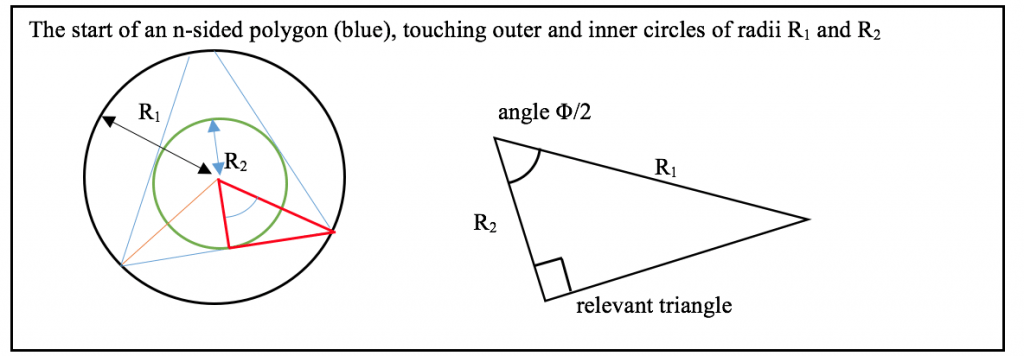# Between a square rock and a hard pentagon: fractional polygons

A polygon with four and a half sides?!Image: Adapted from Flickr user Mélisande*, CC BY-NC-SA 2.0

It wasn’t long after I had dabbled with negative sided polygons (see Thinking outside the box), that I wondered if I could venture outside another geometrical limit: that of non-integer sided polygons!

[Waits for sharp intake of breath. Pressure drops and ears pop.]

Well, why not? If we take the number line and agree that it went (not exactly in this order) from a simple set of positive integers, the positive counting numbers, to include zero and the negative integers, we can draw a parallel to the number of sides of a polygon, as we have now added the negative integer polygons to the positive ones. To our number line of all integers, we then added fractions; all those rational numbers made up of one integer divided by another integer, such as $3/2$ or $1/4$ or $-22/7$ and these filled up many of the gaps between the whole numbers. Maybe we could do the same with polygons?

I did wonder if, like the real number line, there would be a continuum of polygon types—a complete spectrum so to speak, from the visible polygons (the positive ones) to include the anti-polygons (the negative ones… how about anti-gons for a name?) and intra-polygons (the ones in-between, maybe intragons is the word). Oooh! I feel new terminology coming on—I’d better sit down. There, that’s better.Image: Hugh Duncan

Let’s call the fractional-sided shapes fractogons. I like that. I know this would probably have the ancient Greeks turning in their graves, but who knows what genies might pop into mathematical existence if we carry on rubbing this fractionally-sided lamp!

When I started this line of inquiry, I didn’t know that non-integer sided polygons had already been considered and had already been known (but under a different name) for thousands of years. I thought I was being quite a rebel, throwing aside the established rules and boldly going where no one had boldly gone before—to the dark side so to speak. In the spirit of a mathematical explorer, I will share with you my own personal journey.

It all started after Christmas Day celebrations in 2009. We’d had the usual family meal, which ended as most yuletide family dinners do, by playing games-in this case the name game. I was given the Post-it on the forehead with the name Mr Bean, which took me ages to get and I blamed the unsure ‘yes–no’ answers and not my alcohol levels. Anyway, having had my fill of food and social interaction for one day, I sat and pondered. I had recently been working on several maths ideas, such as extraneous triangles (a mix of positive and negative sides—I can tell you about them another time) and negative dimensions (less than a point! Those too!). I ended up reflecting on the negative polygons idea (which was to be published 8 years later) when suddenly I had another lightbulb moment. I actually mentioned it in my diary at the time:

‘I was even wondering if I should extend the $(n-2)180°$ formula from not only the positive integers and negative integers, but how about fractions?! Like half a side?! If $n = 3.5$, the angle inside would be $270°$, but how could one draw such a shape? Oooh, I feel another possible weird branch joining the others!’

We started last time with the equation that gives the total internal angle $\theta$ for an $n$-sided polygon, $n$ of course originally meaning a positive integer:

$$\theta = (n-2)180°.$$

Now, another way of drawing a regular polygon (let’s stick to regular ones for now), is to know the external angle, $\phi$, which is the angle one turns through from the first side before drawing the next side (it is also the same as the angle subtended by one side as seen from the centre). That angle, $\phi$, with $n$ the number of sides, is given by

$$\phi = 360°/n.$$

Take the square as an example, where $n = 4$. That gives an external angle of $\phi = 360°/4 = 90°$. So to draw a square, one draws the first side. Let’s make it horizontal, going right (see below). At the end of that line, imagine extending a dotted line in the same direction and imagine looking along it. Now turn your gaze through $90°$ turning left (that is anti-clockwise, to stick with the mathematical convention). Now draw the second line. Repeat this at the end of the second line and so on until you get back to where you started. You have basically made four left turns, as if you were driving ‘round the block’.Image: Hugh Duncan

Voila, you have drawn a square. Notice, we stopped adding sides when the end of the fourth side joined up exactly to the start of the first side. That makes four sides in one complete turn, which we could write as four sides per turn or $4/1$, which is simply 4. There is a reason for this style of approach, which will become clear shortly. We could have carried on adding sides, couldn’t we, but they would have just laid superimposed on top of the first four, before we got back to the start again. Had we done that, we would have drawn eight sides, but we would have gone twice ‘round the block’. The shape would still have looked the same; a square.

If we write this information as $(\text{number of sides})/(\text{number of turns})$, we get $8/2$. This is like a fraction, which simplifies to $4/1$ or just four, stating that the shape has four sides per turn, as we did with the ‘one turn’ square. We could have gone round a third time and drawn four more sides, and they would have sat on the previous 8, so we would have a total of 12 sides in three turns. In fraction form that’s $12/3$ which again simplifies to $4/1$ or four sides per turn. It is worth bearing in mind this way of constructing polygons, as it will make it easier to find a meaning for fractional sided shapes.

What about drawing a pentagon? Similar process. The external angle is given by

$$\phi = 360°/n,$$

and as $n = 5$ for a pentagon, you get

$$\phi = 360°/5 = 72°.$$

So to draw our pentagon we start with the first edge, extend a dotted line, then turn $72°$ anticlockwise and draw the second, then $72°$ again and the third and so on until the fifth side which joins nicely and neatly back onto the very first point of the first side (see below). We have five sides in one turn, or $5/1$ or just 5 sides. As with the square, we could go round again and draw five more sides before we get back to the start, hence making ten sides in two turns or $10/2$ which becomes $5/1$ or five sides per turn. You see where this is leading?Image: Hugh Duncan

This is middle school maths I know. But now we are going to read between the lines! I asked myself, what would a four and a half sided polygon look like? I had been celebrating Christmas, remember and though I can do some mathematical things in my head, I could not picture what a $4 \frac{1}{2}$-sided polygon would look like-certainly not after all that eating, drinking and being Mr Bean! I had an image in my head that it would resemble an Epicyclical Spiral of Theodorus (look it up!), but that was not quite right. A few days later, I took a sharp pencil, ruler, protractor and a sheet of paper and drew it.

For an $n$-sided polygon, the total internal angle $\theta$ is given by

$$\theta = (n-2)180°.$$

For the traditional polygons, $n$ is an integer greater than or equal to three, while for the unconventional polygons that we met last time, $n$ is an integer less than three. Well, we actually concentrated on it being an integer that was negative two or below. Now we are considering that $n$ can be any rational number! Let us take that simple example, between a square and a pentagon. I called this $4 \frac{1}{2}$-sided polygon a quadrodemigon as it sounded a little mystical. I worked out that its total internal angle would be

$$\theta = 180°(4.5 – 2) = 2.5 \times 180° = 450°.$$

The big question was: what would it look like, so I drew it. Seen as an angle from the centre of the polygon, each side would subtend an angle given by

$$360°/4.5 = 80°,$$

which is also the external angle. The internal angle for each side would be

$$180° – \text{external angle} = 180° – 80° = 100°.$$

Drawing the shape as a regular $4 \frac{1}{2}$-sided polygon means that each side will have the same length and each vertex will contain the same angle: $100°$. See below the first four sides drawn on the left.Image: Hugh Duncan

Note first that four sides do no complete the shape. However, five sides go too far and the first and fifth sides overlap but do not meet up to create a closed shape.

With the square, it became complete when the end of the final edge coincided with the first point of the first edge. If this is how one can define a complete polygon, then more sides should be added to the first five sides of our $4 \frac{1}{2}$-sided shape until the last point of the last side meets with the first point of the first side. In this case (and as will be seen, in all future non-integer cases) this would mean allowing sides to overlap and cross.

Continuing to add sides following this rule, one finds that the $4 \frac{1}{2}$-sided shape becomes closed after a total of nine sides and it has taken two complete turns. Here, the final point of the ninth side meets up with the first point of the first side. The shape is a nine-pointed ‘star’ (see below left)!Image: Hugh Duncan

If the same approach (sides per turn) is taken for all polygons, then our nine-sided star taking two turns would have $9/2$ sides per turn. Thus $9/2 = 4.5$. So perhaps this is what is meant by a fractional-sided polygon. As the total internal angle was $900°$,  $( 9 \times 100°)$ but that was over two turns, then that would work out to be $900°/2 = 450°$ per turn. This agrees with the total internal angle given by the original equation. With these fractional-sided polygons already known, some have questioned whether this $9/2$ sided shape is really a $4 \frac{1}{2}$-sided polygon, or in fact two $4 \frac{1}{2}$-sided polygons stuck together. Perhaps that’s a question for the mathematical philosophers.

## Alive and kicking for years

I eventually found out that this $4 \frac{1}{2}$-sided shape was already known and called a nonangle and was an example of what are called crossed or self-intersecting polygons. This was when I acquired a copy of ‘Regular Polytopes’ by Coxeter-a fascinating look into higher dimensional shapes. There are some even more famous and well-known crossed polygons than the nonangle. Let’s look at other odd half-integer sided shapes, for example, a $5 \frac{1}{2}$ sided polygon before we see the most famous of them all. Our $5 \frac{1}{2}$-sider would have 11 full sides in two complete turns, before closing the shape and it will contain a total internal angle of:

$$\theta = (11-2)180°,$$

for two turns, which is $1620°/2 = 810°$ per turn. This would be an eleven-pointed star like the nine-pointed nonangle (see below left—note I have left it shaded in the way the Microsoft Word shape-drawing program chooses. More about that later). This approach will also work for shapes with a smaller number of odd half-integer sides, e.g. $3 \frac{1}{2}$, which will become a 7 pointed star over two turns called a heptangle (see below middle). A $2 \frac{1}{2}$ sided polygon will become the well-known 5 pointed star called a pentangle or pentagram (see below right). In fact, all odd half-integer multiple-sided polygons will be completed in two turns.Image: Hugh Duncan

The pentagram is the very shape that, according to Wikipedia, appeared in 3000BC on a Babylonian stone!
If one was to consider a shape with say, $3 \frac{1}{3}$ sides, it would need three turns to complete the shape, creating ten sides $(3 \times 3 \frac{1}{3} = 10$ and this would look like a ten-pointed star. It could be called a $3.333333\dots$ sided polygon, or $10/3$ or possibly given the name decangle (this is shown later). It should be obvious that the number of turns needed to complete a fractional sided polygon depends on the fractional part, the number of turns being equal to the denominator of the fraction part, hence $4 \frac{1}{2}$ sides needs $1/0.5 = 2$ turns, while a $4.333333$ sider needs $1/0.333333 = 3$ turns and a $4.25$ sider needs $1/0.25 = 4$ turns. A shape with say, $3.11$ sides will need (as it is 3 and $11/100$) 100 turns, some 311 points on that star!

## Negative fractional sided polygons

As for the negative sided versions of these fractogons, one need only follow the same reasoning as before. Take the pentagram, where there is $360° – 36° = 324°$ outside each corner. This would mean $-324°$ for the anti-pentagram. There would be five sides in two turns so $-324° \times 5 = -1620°$ or $-810°$ in one turn. Using the formula with $n = -2.5$ gives

$$180° (-2.5 – 2) = 180° \times (-4.5) = -810°,$$

which agrees with what was expected. This would just be a pentagram-shaped hole or anti-pentagram. The same would follow for any other negative fractogon.

The question now is, are there everyday examples of these fractional sided polygons? Well, of course, there are! Though under different names. See below some examples:Image: Hugh Duncan

As if that wasn’t enough, let us just zoom in to have a closer look at some of the fractional sided polygons that can be found between the digon (2 sides) and the $3 \frac{1}{3}$ sider. More stars and flowers! There are of course an infinite number of these interlopers, but I have chosen a selection of those with smaller sized denominators.Image: Hugh Duncan

To complete the tour, here is a close-up of the equivalent range among the negative polygons. Some remind me of the nozzles of cream syringes or cupcake pastry cutters. Like I said—everywhere…Image: Hugh Duncan

## Definition of crossed (self-intersecting) polygons

Wolfram gives the definition of crossed polygons in the following way. They are identified by two positive integers in the form of a fraction $p/q$, such that $p$, the numerator, is the number of points on a circle (evenly spaced for regular shapes), and $q$, the denominator, indicates the spacing between points joined by an edge. So for example, $5/2$ means that you draw five points equidistant on the circumference of a circle and you join every second point. This gives the well-known five-pointed star or pentagram. See below. When one changes the values of p and q to $6/2$, it means you draw six evenly spaced points on the circle and you join together every second one. Now you get an equilateral triangle first time round, then a second one the second time round, but upside down compared to the first (or one could say displaced rotationally by $60°$). The end result is two equilateral triangles that cross and make the six-pointed star more commonly known as the Star of David. See below. Similarly, a crossed polygon of the form $8/2$ would be made from eight points in a circle and joining every second one. This creates two squares that are displaced one from the other rotationally by $45°$. See in the diagram later on.Image: Hugh DuncanImage: Hugh Duncan

The Chalkdust reader may have spotted that these ‘odd’ sided crossed polygons give the same result as the odd sided fractogons ($5/2$, $7/2$, $9/2$ etc) but the ‘even’ sided crossed polygons do not match up with the even sided fractogons. The $6/2$ fractogon would merely give an equilateral triangle (actually two, but one drawn exactly on top of the other), while the crossed polygon version gives the two mirror image equilateral triangles that make the six-pointed Star of David. The $8/2$ fractogon would just be one square superimposed upon a second, after having been drawn by going round twice, while the $8/2$ crossed polygon is made of two separate squares, displaced by $45°$ to generate the 8 pointed octangle. Thus the two ways of looking at the polygons between the integer sided shapes are slightly different. One could represent each set on a Venn diagram, showing the common shapes in the ‘intersection’ area with the different interpretations remaining outside the overlap. So perhaps there is an acknowledgeable difference between the fractogon interpretation (fractionally-sided polygons) and the generally accepted crossed (self-intersecting) ones. What do you think?Image: Hugh Duncan

## A transcendental polygon: the piangle

Now that we have filled in all the rational polygons, whatever gaps still remain must be the irrational and transcendental polygons. Let’s just look at one in detail. I have decided to choose none other than a polygon with the same number of sides as that most meditative of transcendentals: pi. Oh yes! And why not call it a piangle while we’re at it?! Or a pi-gon?! It will have about 3.14159 sides in each turn. What will it look like? Let us start the operation. Nurse, the angle formula please:

$$\phi = 360°/\pi = 114.59\dots°.$$

As seen from the centre, each side will subtend $114.59\dots°$. Three sides will almost complete the circle but fall a tiny bit short (see below left). Keep adding sides and 22 sides will be created in a smidgen over seven turns (the fraction $22/7$ would fit perfectly-see below middle left). Continue adding sides until boredom kicks in-for me it was after 71 of them (see middle right). Note, one can start to see the inner and outer circular limits to the dimensions of the pi-gon, traced out by the edges.Image: Hugh Duncan

One would then have to keep on adding sides without end, as our pi-gon will never meet up with the starting point. Thus we would get so many overlapping edges that a huge ring-shaped space will be taken up by all these edges (see above right), such that the inner radius touched by all the sides is about $0.54$ the size of the outer radius (this is calculated below). It is curious that a piangle looks like a circular disc, but if all non-rational polygons are considered, all of them would end up looking like ringed areas, with the inner radii that the sides touch being a certain fraction of the size of the outer radii, given by the formula below. This is because all irrationals and transcendentals do not repeat in their decimal expansions. So, the spaces between the finite sided shapes would be filled with these infinite sided blurred rings, all due to the inclusion of the irrationals and transcendentals.

There would be a similar filling-in of the anti-polygons on the negative side of the polygonal continuum, with the anti-irrational polygons and anti-transcendentals filling in all the gaps between the anti-rationals. It is possible to show the sizes of the inner and outer rings of these ‘poly-fillers’ by a little bit of trigonometry:Image: Hugh Duncan

The angle subtended by one side as seen from the centre of the shape, $\phi$, is given by $\phi = 360/n$ where $n$ is the number of sides of the polygon. Looking at the diagram above, the outer points of the polygon touch a circle of radius $R_1$ while the middle of the edges touch a smaller inner circle of radius $R_2$. The two radii are part of the same right-angled triangle with $R_1$ the hypotenuse and the common angle is, in fact, half the angle $\phi$.

As $\text{adjacent}/\text{hypotenuse} = \text{cosine}$, then $R_2/R_1 = \cos(\phi /2)$.

As $\phi = 360°/n$ then substituting in for $\phi$ gives

$$R_2/R_1 = \cos(360°/2n) = \cos(180°/n).$$

As an example, for a square $R_2/R_1 = \cos (180°/4) = 1/\sqrt{2} = 0.7071$. This means that the inner circle touched by the sides of the square is $0.7071$ the radius of the outer circle.

For an equilateral triangle $R_2/R_1 = \cos (180°/3) = \cos(60) = 0.5$. Thus the inner circle touched by the sides is half the radius of the outer circle.

So, for a piangle, $R_2/R_1 = \cos(180°/\pi) = \cos (57.3°) = 0.5403.$

We have now completely filled the polygonal continuum from $n = 2$ and above and from $n = -2$ and below. If the maths police don’t arrest me and lock me away, then perhaps next time we can have a look inside that embarrassing gap that I’ve left and which has been staring back saying: ‘what about us? Not enough sides to worry about?’ What does lie between $n = 2$ and $n = -2$?

## Post script: the polygonal quarks of beauty

There is a strong, loving link between maths and art and, just as a little side step so we can stop and smell the wild polygonal flowers, I wanted to show you a creative way of representing these fractogons. Microsoft Word has a shape drawing application that allows you to draw the construction lines of these crossed polygons and it colours the different areas with one of two colours, depending on whether that area is inside or outside the closed shape. Then by adding suitable shadowing, they can be shown to be either positive or negative fractogons. I reproduce a selection of them below for your enjoyment:Just as the vacuum of empty space is teaming with countless virtual particles, that come and go in the blink of an eye, perhaps our polygonal number line is similarly populated with all these virtual shapes between the integers. Exotic particles of the mathematical universe if you like. As we have got down to the two-sided digon and filled in all the gaps, perhaps next time we will be ready to go below two sides and uncover the next layer: the shapes I call overturned polygons…

Welcome to the under side of mathematics!Hugh Duncan graduated from UCL in 1980 having studied astronomy. He teaches physics and maths in the International School of Nice. Hugh was a punk when he started UCL in 1976 and guess what, he still is! Check out his band Old Age Spies on YouTube.
+ More articles by Hugh

## 2 thoughts on “Between a square rock and a hard pentagon: fractional polygons”

1.Daniele says:

Hi, I’ve thoght about this since some years. I’ve also faced the analogy with complex root of unity. In this context one can imagine , for example, the equation x^(5/3)=1 to have exactly 5/3 solutions, where the solutions could represent the verteces of fractogon 5/3. The point is “what do it means” to have 5/3 solutions or 5/3 verteces?

2.Stephen Nurse says:

Hi, This article got me thinking as well, or rather saved me from thinking! Anyway, click through to my blog at http://tribo3d.blogspot.com/2019/04/rigid-spiral-from-thingiverse-parts.html to see an application for this mathematics, creating a theory as to how some interlocking parts become rigid when assembled in a special way. Thanks for Hugh Duncan for the original article and I I will try to get my head around Daniele’s comment!

Regards

Steve nurse Melbourne Australia